Download Presentation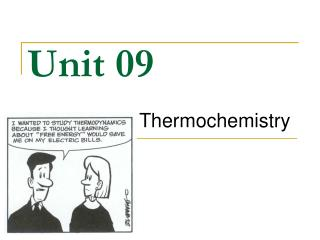Unit 09

# Unit 09 - PowerPoint PPT Presentation

Unit 09. Thermochemistry. Unit 9 Vocabulary. Thermochemistry - concerned with heat changes that occur during chemical reactions Energy - capacity of an object to do work or produce heat Kinetic energy - the energy an object has due to its motion Potential energy - stored energyI am the owner, or an agent authorized to act on behalf of the owner, of the copyrighted work described.
Download Presentation## Unit 09

An Image/Link below is provided (as is) to download presentation

Download Policy: Content on the Website is provided to you AS IS for your information and personal use and may not be sold / licensed / shared on other websites without getting consent from its author.While downloading, if for some reason you are not able to download a presentation, the publisher may have deleted the file from their server.

- - - - - - - - - - - - - - - - - - - - - - - - - - E N D - - - - - - - - - - - - - - - - - - - - - - - - - -
Presentation Transcript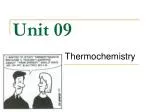### Unit 09

Thermochemistry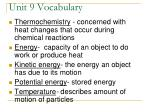Unit 9 Vocabulary
• Thermochemistry - concerned with heat changes that occur during chemical reactions
• Energy- capacity of an object to do work or produce heat
• Kinetic energy- the energy an object has due to its motion
• Potential energy- stored energy
• Temperature- describes amount of motion of particles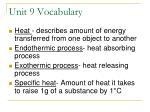Unit 9 Vocabulary
• Heat - describes amount of energy transferred from one object to another
• Endothermic process- heat absorbing process
• Exothermic process- heat releasing process
• Specific heat- Amount of heat it takes to raise 1g of a substance by 1°C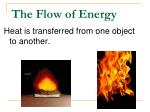The Flow of Energy

Heat is transferred from one object to another.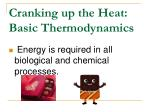Cranking up the Heat: Basic Thermodynamics
• Energy is required in all biological and chemical processes.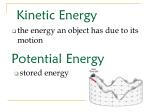Kinetic Energy
• the energy an object has due to its motion

Potential Energy

• stored energy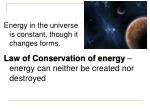Energy in the universe is constant, though it changes forms.

Law of Conservation of energy – energy can neither be created nor destroyed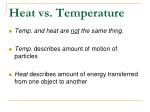Heat vs. Temperature
• Temp. and heat are not the same thing.
• Temp. describes amount of motion of particles
• Heat describes amount of energy transferred from one object to another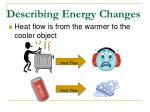Describing Energy Changes

Heat flow is from the warmer to the cooler object

Heat Flow

Heat Flow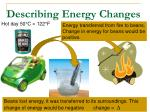Describing Energy Changes

Hot day 50°C = 122°F

Energy transferred from fire to beans. Change in energy for beans would be positive.

Beans lost energy, it was transferred to its surroundings. This change of energy would be negative change = ∆Endothermic reactions – absorbs heat, ∆E is positive, the surroundings cool down

Exothermic reactions – releases heat, ∆E is negative, the surroundings heat up

Surroundings

Heat

Surroundings

Describing Energy Changes

HeatUnits used to describe Energy:

calorie – called “little calorie” – (cal)

kilocalorie – called “Big calorie”- (CAL)

1000 calories – same as a food calorie

Joule – the SI unit of heat - (J)CONVERSION FACTOR

1 cal = 4.184 joules

1 cal 4.184 J

4.184 J 1 calHeat Units: Conversion Problems

1. Convert 32.7 cal to joules.

2. Convert 6.24 J to cal.Specific Heat -

Amount of heat it takes to raise 1g of a substance by 1°C

Metals have a low specific heat

Water has a high specific heatLearning Check

1. On a sunny day, the concrete deck around an outdoor swimming pool becomes hot, while the water stays cool. This is because…

A. The deck has a higher specific heat than the water

B. The deck has a lower specific heat then the water

C. Both objects have the same specific heatLearning Check

2. Two objects are sitting next to each other in the sunlight. Object A gets hotter than object B.

A. Object A has a lower specific heat than object B

B. Object A has a higher specific heat than object B

C. Both objects have the same specific heatSpecific Heat of Water

4.18 J/g ×°C

or

1 cal/g ×°C

Matches definition: Amount of heat it takes to raise 1g of a substance by 1°CFormula for Specific Heat

q = amount of heat – measured in cal or J

m = mass – measured in g

C = specific heat – units are J/g x °C or cal/g x °C

ΔT = change in temp – measured in °C

q = mc ΔTSpecific Heat Problems:

1. Aluminum has a specific heat of 0.9020 J/g × °C. How much heat is lost when a piece of aluminum with a mass of 23.984 g cools from a temperature of 415.0°C to a temperature of 22.0°C?

2. 850 calories of heat are applied to a 250 g sample of liquid water with an initial temperature of 13.0 °C.

Find: a) the change in temperature and

b) the final temperature.

(remember, the specific heat of liquid water, in calories, is 1.00 cal/g x °C.)3. The temperature of a sample of water increases by 69.5 °C when 24,500 J are applied.  The specific heat of liquid water is 4.18 J/g x °C.  What is the mass of the sample of water?

4. When 34,700 J of heat are applied to a 350 g sample of an unknown material the temperature rises from 22.0°C to 173.0°C.  What must be the specific heat of this material?

Specific Heat Problems:Bell Work - Wed 1/23/08

When 34,700 J of heat are applied to a 350 g sample of an unknown material the temperature rises from 22.0°C to 173.0°C.  What must be the specific heat of this material?Energy and Change of State

You do not have to write this

When energy is added to a solid substance, its temperature increases until its melting point is reached. Upon the addition of more energy, the substance begins to melt.

 The temperature remains the same until all the substance has melted. ****Enthalpy of Fusion (DHfus)

Heating Curve: ( For Water)

Temp

DHfus

Melting point

(°C)

0

-10

Heating Ice

(solid)

-20

TimeAfter the substance melts and if heating continues, the temperature will continue to rise until it reaches its boiling point.

۞The temperature remains the same until all the substance has boiled.***

Energy and Change of StateEnthalpy of Vaporization (DHvap)

Boiling Point

100

DHfus

DHvap

Temp

Heating Water

(liquid)

Melting Point

0

(°C)

-10

Heating Ice

-20

Time

Energy required to vaporize 1 gram of a substance at its boiling point► Important Formulas to Know ◄

When a substance is being heated, use the following formula: (inclined line)

q = (m)(c)(DT)

When a substance is melting or boiling, use the following formula: (flat line)

q = (m) (DHfus or DHvap)q = (m)(c)(DT)

(C) = Specific Heat:

- of ice = 2.06 J/g x °C

- of water = 4.18 J/g x °C

- of steam = 2.02 J/g x °Cq = (m) (DHfus or DHvap)

Enthalpy Values for Water:

DHfus = 334 J/g

DHvap = 2260 J/gSample Problem #1

How much heat is required to melt 5.67g of ice?

Q = (m)(ΔH fus)

Q = (5.67g)(334 J/g)

Q =Sample Problem #2

How much energy is required to boil 85g of water?Sample Problem #3

How much energy is required to raise the temperature of 25g of water from 15°C to 75°C ?Sample Problem #4

How much energy is required to convert 40g of ice at -10°C to steam at 150°C ?

Use Both Formulas

Draw a graphSample Problem #5

What is the final temperature when 1250 J of energy is added to 15g of water at 25°C ?Warm Up

How much energy is required to boil 50 grams of water?

What is the final temperature when 2500 J of heat is added to 35 grams of water at 15 degrees Celsius?

How much energy is required to convert 75 grams of ice at -20 degrees Celsius to steam at 125 degrees Celsius? (Hint: This problem has 5 steps!!)